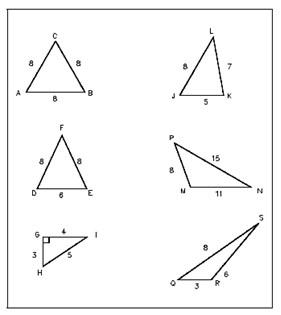## Triangles Assignment Help

Assignment Help: >> Shapes and Figures of Plane Geometry - Triangles

Triangles:

A triangle is a figure created by using straight line segments to connect three points which are not in a straight line. The straight line segments are known as sides of the triangle.

Examples of a number of kinds of triangles are shown in Figure.  An equilateral triangle is one in that all three sides and all three angles are equal. Triangle ABC in Figure is an example of an equilateral triangle. An isosceles triangle has two equal sides & two equal angles (triangle DEF). A right side triangle has one of its angles equal to 90° and is the most significant triangle for our studies (triangle GHI).  An acute triangle has every of its angles less than 90° (triangle JKL). Triangle MNP is known as a scalene triangle because each side is a different length.  Triangle QRS is to be considered an obtuse triangle since it has one angle greater than 90°.  A triangle may have more than one of these attributes.  The total sum of the interior angles in a triangle is always 180°.Figure: Types of Triangles#### Assured A++ Grade

Get guaranteed satisfaction & time on delivery in every assignment order you paid with us! We ensure premium quality solution document along with free turntin report!